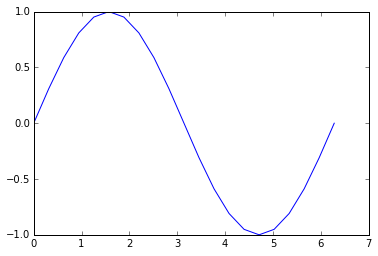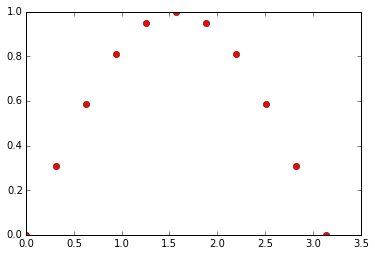# Numpy 简介¶

## 导入numpy¶

NumpyPython的一个很重要的第三方库，很多其他科学计算的第三方库都是以Numpy为基础建立的。

Numpy的一个重要特性是它的数组计算。

In :
from numpy import *


import numpy
import numpy as np
from numpy import *
from numpy import array, sin


In :
%pylab

Using matplotlib backend: Qt4Agg
Populating the interactive namespace from numpy and matplotlib


## 数组上的数学操作¶

In :
a = [1, 2, 3, 4]
a + 1

---------------------------------------------------------------------------
TypeError                                 Traceback (most recent call last)
<ipython-input-3-068856d2a224> in <module>()
1 a = [1, 2, 3, 4]
----> 2 a + 1

TypeError: can only concatenate list (not "int") to list

In :
a = array(a)
a

Out:
array([1, 2, 3, 4])

array 数组支持每个元素加 1 这样的操作：

In :
a + 1

Out:
array([2, 3, 4, 5])

In :
b = array([2, 3, 4, 5])
a + b

Out:
array([3, 5, 7, 9])

In :
a * b

Out:
array([ 2,  6, 12, 20])

In :
a ** b

Out:
array([   1,    8,   81, 1024])

## 提取数组中的元素¶

In :
a

Out:
1

In :
a[:2]

Out:
array([1, 2])

In :
a[-2:]

Out:
array([3, 4])

In :
a[:2] + a[-2:]

Out:
array([4, 6])

## 修改数组形状¶

In :
a.shape

Out:
(4L,)

In :
a.shape = 2,2
a

Out:
array([[1, 2],
[3, 4]])

## 多维数组¶

a 现在变成了一个二维的数组，可以进行加法：

In :
a + a

Out:
array([[2, 4],
[6, 8]])

In :
a * a

Out:
array([[ 1,  4],
[ 9, 16]])

## 画图¶

linspace 用来生成一组等间隔的数据：

In :
a = linspace(0, 2*pi, 21)
%precision 3
a

Out:
array([ 0.   ,  0.314,  0.628,  0.942,  1.257,  1.571,  1.885,  2.199,
2.513,  2.827,  3.142,  3.456,  3.77 ,  4.084,  4.398,  4.712,
5.027,  5.341,  5.655,  5.969,  6.283])

In :
b = sin(a)
b

Out:
array([  0.000e+00,   3.090e-01,   5.878e-01,   8.090e-01,   9.511e-01,
1.000e+00,   9.511e-01,   8.090e-01,   5.878e-01,   3.090e-01,
1.225e-16,  -3.090e-01,  -5.878e-01,  -8.090e-01,  -9.511e-01,
-1.000e+00,  -9.511e-01,  -8.090e-01,  -5.878e-01,  -3.090e-01,
-2.449e-16])

In :
%matplotlib inline
plot(a, b)

Out:
[<matplotlib.lines.Line2D at 0xa128ba8>]## 从数组中选择元素¶

In :
b >= 0

Out:
array([ True,  True,  True,  True,  True,  True,  True,  True,  True,
True,  True, False, False, False, False, False, False, False,
False, False, False], dtype=bool)
In :
mask = b >= 0


In :
plot(a[mask], b[mask], 'ro')

Out:
[<matplotlib.lines.Line2D at 0xa177be0>]